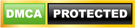Blog

• Home
• LabVIEW
• LABVIEW – Digital Waveform Graphs And Data Type

# LABVIEW – Digital Waveform Graphs And Data Type

## Digital Waveform Graphs

Use the digital waveform graph to display digital data, especially when you work with timing diagrams or logic analyzers. The digital waveform graph accepts the digital waveform data type, the digital data type, and an ARRAY of those data types as an input. By default, the digital waveform graph collapses digital buses, so the graph plots digital data on a single plot. If you wire an array of digital data, the digital WAVEFORM GRAPH plots each element of the array as a different plot in the order of the array.

The digital waveform graph in Figure (a) shows the FRONT PANEL plots digital data on a single plot. The VI converts the numbers in the Numbers array to digital data and displays the binary representations of the numbers in the Binary Representations digital data indicator. In the digital graph, the number 0 appears without a top line to symbolize that all the bit values are zero. The number 255 appears without a bottom line to symbolize that all the bit values are one. Right-click the y-scale and select Expand Digital Buses from the shortcut menu to plot each sample of digital data. Each plot represents a different bit in the digital pattern. The digital waveform graph in Figure (b) shows the front panel displays the six numbers in the Numbers array.

Enhance your IT skills and proficiency by taking up the LabVIEW Training.(A)(B)

The Binary Representations digital indicator displays the binary representations of the numbers. Each column in the table represents a bit. For example, the number 89 requires 7 bits of memory (the 0 in column 7 indicates an unused bit). Point 3 on the digital waveform graph plots the 7 bits necessary to represent the number 89 and a value of 0 to represent the unused eighth bit on plot 7. The VI in the below Figure converts an array of numbers to digital data and uses the Build Waveform function to assemble the start time, delta t, and the numbers entered in a digital data control and to display the digital data.## Digital Waveform Data Type

The digital waveform data type carries start time, delta x, the data and the attributes of a digital waveform. You can use the Build Waveform function to create a digital waveform. When you wire digital waveform data to the digital waveform graph, the graph automatically plots a waveform based on the timing information and data of the digital waveform. Wire digital waveform data to a digital data indicator to view the samples and signals of a digital waveform.

Check Out LabVIEW Tutorials